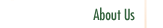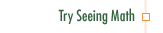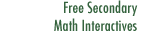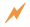Quadratic Equations

In Quadratic Equations, we make the relationship between quadratic functions and quadratic equations explicit. Because textbooks and tests devote a great deal of time to the skills of factoring and finding roots, participants also use graphical means, as well as successive approximations in tabular form, to reach the same goal.

Professional Development Goals

• Identify the three symbolic forms for quadratic equations—polynomial, product, and vertex—and clarify how each form's role informs not only its graphic representation, but how to find the root of its function
• Delineate techniques for solving quadratic equations by comparing quadratic functions, both graphically and symbolically
• Solve quadratic inequalities, in both graphic and symbolic forms, as a natural extension of comparing functionsClick to Get Seeing Math™ SecondaryCourse Listing Proportional Reasoning Linear Functions Transformations of Linear Functions Linear Equations Systems of Linear Equations Quadratic Functions Transformations of Quadratic Functions Quadratic Equations Data Analysis